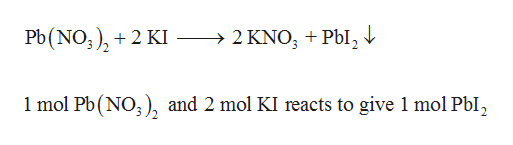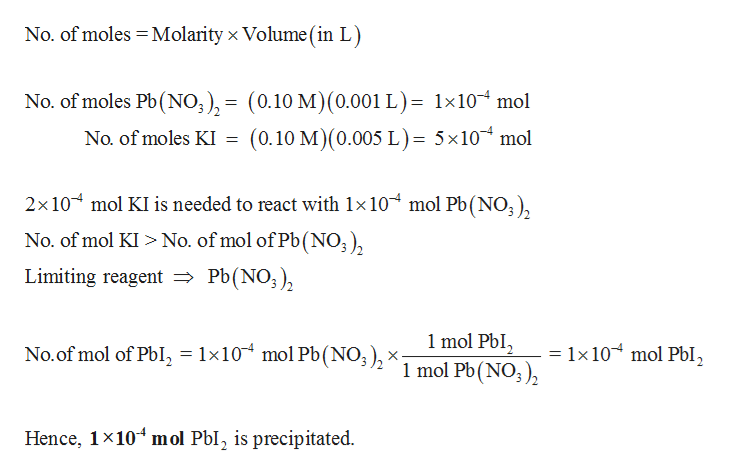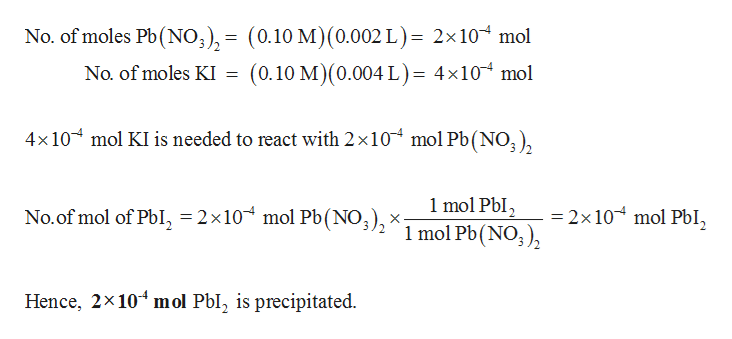# Calculate the number of moles of precipitate that would be produced when two solutions were mixed. a) 1.0 mL 0.10 M Pb(NO3)2 and 5.0 mL, 0.10 M KIb) 2.0 mL 0.10 M Pb(NO3)2 and 4.0 mL, 0.10 M KIc) 3.0 mL 0.10 M Pb(NO3)2 and 3.0 mL, 0.10 M KId) 4.0 mL 0.10 M Pb(NO3)2 and 2.0 mL, 0.10 M KIe) 5.0 mL 0.10 M Pb(NO3)2 and 1.0 mL, 0.10 M KI

Question
8 views

Calculate the number of moles of precipitate that would be produced when two solutions were mixed.

a) 1.0 mL 0.10 M Pb(NO3)2 and 5.0 mL, 0.10 M KI

b) 2.0 mL 0.10 M Pb(NO3)2 and 4.0 mL, 0.10 M KI

c) 3.0 mL 0.10 M Pb(NO3)and 3.0 mL, 0.10 M KI

d) 4.0 mL 0.10 M Pb(NO3)2 and 2.0 mL, 0.10 M KI

e) 5.0 mL 0.10 M Pb(NO3)2 and 1.0 mL, 0.10 M KI

check_circle

Step 1

PbI2 is precipitated when Pb(NO3)2 and KI.help_outlineImage TranscriptionclosePb(NO),2 KI >2 KNO3 +Pbl2 1 mol Pb(NO), and 2 mol KI reacts to give 1 mol Pbl, fullscreen
Step 2

(a)

Number of moles of precipitate (PbI2) formed is calculated as follows,

1 mL = 0.001 Lhelp_outlineImage TranscriptioncloseNo. of moles Molarity x Volume (in L) No. of moles Pb (NO), = (0.10 M) (0.001 L)= 1x104 mol (0.10 M) (0.005 L) = 5x10 mol No. of moles KI 2x10 mol KI is needed to react with 1x 10 mol Pb(NO3) No. of mol KI> No. of mol of Pb(NO) Limiting reagent Pb(NO;), 1 mol PbI 1 mol Pb(NO) = 1x 10 mol PbI No.of mol of Pbl, = 1x10 mol Pb(NO ), Hence, 1x10 mol Pbl, is precipitated. fullscreen
Step 3

(b)

Number of moles of precipitate (PbI2) form...help_outlineImage TranscriptioncloseNo. of moles Pb (NO3), = (0.10 M) (0.002 L)= 2x10 mol (0.10 M) (0.004 L)= 4x10 mol No. of moles KI mol KI is needed to react with 2 x10 mol Pb (NO3) 4x10 1 mol Pbl2 1 mol Pb(NO), 2x 10 mol PbI No.of mol of PbI, = 2 x10 mol Pb(NO3), x- Hence, 2x10 mol Pbl, is precipitated fullscreen

### Want to see the full answer?

See Solution

#### Want to see this answer and more?

Solutions are written by subject experts who are available 24/7. Questions are typically answered within 1 hour.*

See Solution
*Response times may vary by subject and question.
Tagged in

### General Chemistry